# tf.data: Build TensorFlow input pipelines

The tf.data API enables you to build complex input pipelines from simple, reusable pieces. For example, the pipeline for an image model might aggregate data from files in a distributed file system, apply random perturbations to each image, and merge randomly selected images into a batch for training. The pipeline for a text model might involve extracting symbols from raw text data, converting them to embedding identifiers with a lookup table, and batching together sequences of different lengths. The tf.data API makes it possible to handle large amounts of data, read from different data formats, and perform complex transformations.

The tf.data API introduces a tf.data.Dataset abstraction that represents a sequence of elements, in which each element consists of one or more components. For example, in an image pipeline, an element might be a single training example, with a pair of tensor components representing the image and its label.

There are two distinct ways to create a dataset:

• A data source constructs a Dataset from data stored in memory or in one or more files.

• A data transformation constructs a dataset from one or more tf.data.Dataset objects.

import tensorflow as tf

import pathlib
import os
import matplotlib.pyplot as plt
import pandas as pd
import numpy as np

np.set_printoptions(precision=4)


## Basic mechanics

To create an input pipeline, you must start with a data source. For example, to construct a Dataset from data in memory, you can use tf.data.Dataset.from_tensors() or tf.data.Dataset.from_tensor_slices(). Alternatively, if your input data is stored in a file in the recommended TFRecord format, you can use tf.data.TFRecordDataset().

Once you have a Dataset object, you can transform it into a new Dataset by chaining method calls on the tf.data.Dataset object. For example, you can apply per-element transformations such as Dataset.map(), and multi-element transformations such as Dataset.batch(). See the documentation for tf.data.Dataset for a complete list of transformations.

The Dataset object is a Python iterable. This makes it possible to consume its elements using a for loop:

dataset = tf.data.Dataset.from_tensor_slices([8, 3, 0, 8, 2, 1])
dataset

<TensorSliceDataset shapes: (), types: tf.int32>

for elem in dataset:
print(elem.numpy())

8
3
0
8
2
1



Or by explicitly creating a Python iterator using iter and consuming its elements using next:

it = iter(dataset)

print(next(it).numpy())

8



Alternatively, dataset elements can be consumed using the reduce transformation, which reduces all elements to produce a single result. The following example illustrates how to use the reduce transformation to compute the sum of a dataset of integers.

print(dataset.reduce(0, lambda state, value: state + value).numpy())

22



### Dataset structure

A dataset contains elements that each have the same (nested) structure and the individual components of the structure can be of any type representable by tf.TypeSpec, including tf.Tensor, tf.sparse.SparseTensor, tf.RaggedTensor, tf.TensorArray, or tf.data.Dataset.

The Dataset.element_spec property allows you to inspect the type of each element component. The property returns a nested structure of tf.TypeSpec objects, matching the structure of the element, which may be a single component, a tuple of components, or a nested tuple of components. For example:

dataset1 = tf.data.Dataset.from_tensor_slices(tf.random.uniform([4, 10]))

dataset1.element_spec

TensorSpec(shape=(10,), dtype=tf.float32, name=None)

dataset2 = tf.data.Dataset.from_tensor_slices(
(tf.random.uniform(),
tf.random.uniform([4, 100], maxval=100, dtype=tf.int32)))

dataset2.element_spec

(TensorSpec(shape=(), dtype=tf.float32, name=None),
TensorSpec(shape=(100,), dtype=tf.int32, name=None))

dataset3 = tf.data.Dataset.zip((dataset1, dataset2))

dataset3.element_spec

(TensorSpec(shape=(10,), dtype=tf.float32, name=None),
(TensorSpec(shape=(), dtype=tf.float32, name=None),
TensorSpec(shape=(100,), dtype=tf.int32, name=None)))

# Dataset containing a sparse tensor.
dataset4 = tf.data.Dataset.from_tensors(tf.SparseTensor(indices=[[0, 0], [1, 2]], values=[1, 2], dense_shape=[3, 4]))

dataset4.element_spec

SparseTensorSpec(TensorShape([3, 4]), tf.int32)

# Use value_type to see the type of value represented by the element spec
dataset4.element_spec.value_type

tensorflow.python.framework.sparse_tensor.SparseTensor


The Dataset transformations support datasets of any structure. When using the Dataset.map(), and Dataset.filter() transformations, which apply a function to each element, the element structure determines the arguments of the function:

dataset1 = tf.data.Dataset.from_tensor_slices(
tf.random.uniform([4, 10], minval=1, maxval=10, dtype=tf.int32))

dataset1

<TensorSliceDataset shapes: (10,), types: tf.int32>

for z in dataset1:
print(z.numpy())

[1 2 5 3 8 9 6 7 9 3]
[5 4 3 3 9 7 3 1 5 4]
[7 3 4 8 4 8 5 9 4 1]
[8 6 8 2 6 3 5 8 3 3]


dataset2 = tf.data.Dataset.from_tensor_slices(
(tf.random.uniform(),
tf.random.uniform([4, 100], maxval=100, dtype=tf.int32)))

dataset2

<TensorSliceDataset shapes: ((), (100,)), types: (tf.float32, tf.int32)>

dataset3 = tf.data.Dataset.zip((dataset1, dataset2))

dataset3

<ZipDataset shapes: ((10,), ((), (100,))), types: (tf.int32, (tf.float32, tf.int32))>

for a, (b,c) in dataset3:
print('shapes: {a.shape}, {b.shape}, {c.shape}'.format(a=a, b=b, c=c))

shapes: (10,), (), (100,)
shapes: (10,), (), (100,)
shapes: (10,), (), (100,)
shapes: (10,), (), (100,)



### Consuming NumPy arrays

If all of your input data fits in memory, the simplest way to create a Dataset from them is to convert them to tf.Tensor objects and use Dataset.from_tensor_slices().

train, test = tf.keras.datasets.fashion_mnist.load_data()

Downloading data from https://storage.googleapis.com/tensorflow/tf-keras-datasets/train-labels-idx1-ubyte.gz
32768/29515 [=================================] - 0s 0us/step
26427392/26421880 [==============================] - 1s 0us/step
8192/5148 [===============================================] - 0s 0us/step
4423680/4422102 [==============================] - 0s 0us/step


images, labels = train
images = images/255

dataset = tf.data.Dataset.from_tensor_slices((images, labels))
dataset

<TensorSliceDataset shapes: ((28, 28), ()), types: (tf.float64, tf.uint8)>


### Consuming Python generators

Another common data source that can easily be ingested as a tf.data.Dataset is the python generator.

def count(stop):
i = 0
while i<stop:
yield i
i += 1

for n in count(5):
print(n)

0
1
2
3
4



The Dataset.from_generator constructor converts the python generator to a fully functional tf.data.Dataset.

The constructor takes a callable as input, not an iterator. This allows it to restart the generator when it reaches the end. It takes an optional args argument, which is passed as the callable's arguments.

The output_types argument is required because tf.data builds a tf.Graph internally, and graph edges require a tf.dtype.

ds_counter = tf.data.Dataset.from_generator(count, args=, output_types=tf.int32, output_shapes = (), )

for count_batch in ds_counter.repeat().batch(10).take(10):
print(count_batch.numpy())

[0 1 2 3 4 5 6 7 8 9]
[10 11 12 13 14 15 16 17 18 19]
[20 21 22 23 24  0  1  2  3  4]
[ 5  6  7  8  9 10 11 12 13 14]
[15 16 17 18 19 20 21 22 23 24]
[0 1 2 3 4 5 6 7 8 9]
[10 11 12 13 14 15 16 17 18 19]
[20 21 22 23 24  0  1  2  3  4]
[ 5  6  7  8  9 10 11 12 13 14]
[15 16 17 18 19 20 21 22 23 24]



The output_shapes argument is not required but is highly recomended as many tensorflow operations do not support tensors with unknown rank. If the length of a particular axis is unknown or variable, set it as None in the output_shapes.

It's also important to note that the output_shapes and output_types follow the same nesting rules as other dataset methods.

Here is an example generator that demonstrates both aspects, it returns tuples of arrays, where the second array is a vector with unknown length.

def gen_series():
i = 0
while True:
size = np.random.randint(0, 10)
yield i, np.random.normal(size=(size,))
i += 1

for i, series in gen_series():
print(i, ":", str(series))
if i > 5:
break

0 : [1.2226]
1 : [ 0.4785  1.1887 -0.2828  0.6047  1.3367 -0.4387  0.1822]
2 : [ 1.1343 -0.2676  0.0224 -0.111  -0.1384 -1.9315]
3 : [-0.8651]
4 : [0.6275]
5 : [ 0.8034  2.0773  0.6183 -0.3746]
6 : [-0.9439 -0.6686]



The first output is an int32 the second is a float32.

The first item is a scalar, shape (), and the second is a vector of unknown length, shape (None,)

ds_series = tf.data.Dataset.from_generator(
gen_series,
output_types=(tf.int32, tf.float32),
output_shapes=((), (None,)))

ds_series

<FlatMapDataset shapes: ((), (None,)), types: (tf.int32, tf.float32)>


Now it can be used like a regular tf.data.Dataset. Note that when batching a dataset with a variable shape, you need to use Dataset.padded_batch.

ds_series_batch = ds_series.shuffle(20).padded_batch(10)

ids, sequence_batch = next(iter(ds_series_batch))
print(ids.numpy())
print()
print(sequence_batch.numpy())

[18  1  8 22  5 13  6  2 14 28]

[[ 0.0196 -1.007   0.1843  0.0289  0.0735 -0.6279 -1.0877  0.    ]
[ 0.8238 -1.0284  0.      0.      0.      0.      0.      0.    ]
[ 0.544  -1.1061  1.2368  0.3975  0.      0.      0.      0.    ]
[-1.1933 -0.7535  1.0497  0.1764  1.5319  0.9202  0.4027  0.6844]
[-1.2025 -2.7148  1.0702  1.3893  0.      0.      0.      0.    ]
[ 1.3787  0.6817  0.1197 -0.1178  0.9764  0.8895  0.      0.    ]
[-1.523  -0.7722 -2.13    0.2761 -1.1094  0.      0.      0.    ]
[ 0.203   2.1858 -0.722   1.2554  1.2208  0.1813 -2.3427  0.    ]
[ 0.7685  1.7138 -1.2376 -2.6168  0.2565  0.0753  1.5653  0.    ]
[ 0.0908 -0.4535  0.1257 -0.5122  0.      0.      0.      0.    ]]



For a more realistic example, try wrapping preprocessing.image.ImageDataGenerator as a tf.data.Dataset.

flowers = tf.keras.utils.get_file(
'flower_photos',
untar=True)

Downloading data from https://storage.googleapis.com/download.tensorflow.org/example_images/flower_photos.tgz
228818944/228813984 [==============================] - 9s 0us/step



Create the image.ImageDataGenerator

img_gen = tf.keras.preprocessing.image.ImageDataGenerator(rescale=1./255, rotation_range=20)

images, labels = next(img_gen.flow_from_directory(flowers))

Found 3670 images belonging to 5 classes.


print(images.dtype, images.shape)
print(labels.dtype, labels.shape)

float32 (32, 256, 256, 3)
float32 (32, 5)


ds = tf.data.Dataset.from_generator(
lambda: img_gen.flow_from_directory(flowers),
output_types=(tf.float32, tf.float32),
output_shapes=([32,256,256,3], [32,5])
)

ds.element_spec

(TensorSpec(shape=(32, 256, 256, 3), dtype=tf.float32, name=None),
TensorSpec(shape=(32, 5), dtype=tf.float32, name=None))

for images, label in ds.take(1):
print('images.shape: ', images.shape)
print('labels.shape: ', labels.shape)


Found 3670 images belonging to 5 classes.
images.shape:  (32, 256, 256, 3)
labels.shape:  (32, 5)



### Consuming TFRecord data

The tf.data API supports a variety of file formats so that you can process large datasets that do not fit in memory. For example, the TFRecord file format is a simple record-oriented binary format that many TensorFlow applications use for training data. The tf.data.TFRecordDataset class enables you to stream over the contents of one or more TFRecord files as part of an input pipeline.

Here is an example using the test file from the French Street Name Signs (FSNS).

# Creates a dataset that reads all of the examples from two files.

Downloading data from https://storage.googleapis.com/download.tensorflow.org/data/fsns-20160927/testdata/fsns-00000-of-00001
7905280/7904079 [==============================] - 0s 0us/step



The filenames argument to the TFRecordDataset initializer can either be a string, a list of strings, or a tf.Tensor of strings. Therefore if you have two sets of files for training and validation purposes, you can create a factory method that produces the dataset, taking filenames as an input argument:

dataset = tf.data.TFRecordDataset(filenames = [fsns_test_file])
dataset

<TFRecordDatasetV2 shapes: (), types: tf.string>


Many TensorFlow projects use serialized tf.train.Example records in their TFRecord files. These need to be decoded before they can be inspected:

raw_example = next(iter(dataset))
parsed = tf.train.Example.FromString(raw_example.numpy())

parsed.features.feature['image/text']

bytes_list {
value: "Rue Perreyon"
}


### Consuming text data

Many datasets are distributed as one or more text files. The tf.data.TextLineDataset provides an easy way to extract lines from one or more text files. Given one or more filenames, a TextLineDataset will produce one string-valued element per line of those files.

directory_url = 'https://storage.googleapis.com/download.tensorflow.org/data/illiad/'
file_names = ['cowper.txt', 'derby.txt', 'butler.txt']

file_paths = [
tf.keras.utils.get_file(file_name, directory_url + file_name)
for file_name in file_names
]

Downloading data from https://storage.googleapis.com/download.tensorflow.org/data/illiad/cowper.txt
819200/815980 [==============================] - 0s 0us/step
811008/809730 [==============================] - 0s 0us/step
811008/807992 [==============================] - 0s 0us/step


dataset = tf.data.TextLineDataset(file_paths)


Here are the first few lines of the first file:

for line in dataset.take(5):
print(line.numpy())

b"\xef\xbb\xbfAchilles sing, O Goddess! Peleus' son;"
b'His wrath pernicious, who ten thousand woes'
b"Caused to Achaia's host, sent many a soul"
b'And Heroes gave (so stood the will of Jove)'



To alternate lines between files use Dataset.interleave. This makes it easier to shuffle files together. Here are the first, second and third lines from each translation:

files_ds = tf.data.Dataset.from_tensor_slices(file_paths)
lines_ds = files_ds.interleave(tf.data.TextLineDataset, cycle_length=3)

for i, line in enumerate(lines_ds.take(9)):
if i % 3 == 0:
print()
print(line.numpy())


b"\xef\xbb\xbfAchilles sing, O Goddess! Peleus' son;"
b"\xef\xbb\xbfOf Peleus' son, Achilles, sing, O Muse,"
b'\xef\xbb\xbfSing, O goddess, the anger of Achilles son of Peleus, that brought'

b'His wrath pernicious, who ten thousand woes'
b'The vengeance, deep and deadly; whence to Greece'
b'countless ills upon the Achaeans. Many a brave soul did it send'

b"Caused to Achaia's host, sent many a soul"
b'Unnumbered ills arose; which many a soul'
b'hurrying down to Hades, and many a hero did it yield a prey to dogs and'



By default, a TextLineDataset yields every line of each file, which may not be desirable, for example, if the file starts with a header line, or contains comments. These lines can be removed using the Dataset.skip() or Dataset.filter() transformations. Here, you skip the first line, then filter to find only survivors.

titanic_file = tf.keras.utils.get_file("train.csv", "https://storage.googleapis.com/tf-datasets/titanic/train.csv")
titanic_lines = tf.data.TextLineDataset(titanic_file)

Downloading data from https://storage.googleapis.com/tf-datasets/titanic/train.csv
32768/30874 [===============================] - 0s 0us/step


for line in titanic_lines.take(10):
print(line.numpy())

b'survived,sex,age,n_siblings_spouses,parch,fare,class,deck,embark_town,alone'
b'0,male,22.0,1,0,7.25,Third,unknown,Southampton,n'
b'1,female,38.0,1,0,71.2833,First,C,Cherbourg,n'
b'1,female,26.0,0,0,7.925,Third,unknown,Southampton,y'
b'1,female,35.0,1,0,53.1,First,C,Southampton,n'
b'0,male,28.0,0,0,8.4583,Third,unknown,Queenstown,y'
b'0,male,2.0,3,1,21.075,Third,unknown,Southampton,n'
b'1,female,27.0,0,2,11.1333,Third,unknown,Southampton,n'
b'1,female,14.0,1,0,30.0708,Second,unknown,Cherbourg,n'
b'1,female,4.0,1,1,16.7,Third,G,Southampton,n'


def survived(line):
return tf.not_equal(tf.strings.substr(line, 0, 1), "0")

survivors = titanic_lines.skip(1).filter(survived)

for line in survivors.take(10):
print(line.numpy())

b'1,female,38.0,1,0,71.2833,First,C,Cherbourg,n'
b'1,female,26.0,0,0,7.925,Third,unknown,Southampton,y'
b'1,female,35.0,1,0,53.1,First,C,Southampton,n'
b'1,female,27.0,0,2,11.1333,Third,unknown,Southampton,n'
b'1,female,14.0,1,0,30.0708,Second,unknown,Cherbourg,n'
b'1,female,4.0,1,1,16.7,Third,G,Southampton,n'
b'1,male,28.0,0,0,13.0,Second,unknown,Southampton,y'
b'1,female,28.0,0,0,7.225,Third,unknown,Cherbourg,y'
b'1,male,28.0,0,0,35.5,First,A,Southampton,y'
b'1,female,38.0,1,5,31.3875,Third,unknown,Southampton,n'



### Consuming CSV data

The CSV file format is a popular format for storing tabular data in plain text.

For example:

titanic_file = tf.keras.utils.get_file("train.csv", "https://storage.googleapis.com/tf-datasets/titanic/train.csv")

df = pd.read_csv(titanic_file, index_col=None)


If your data fits in memory the same Dataset.from_tensor_slices method works on dictionaries, allowing this data to be easily imported:

titanic_slices = tf.data.Dataset.from_tensor_slices(dict(df))

for feature_batch in titanic_slices.take(1):
for key, value in feature_batch.items():
print("  {!r:20s}: {}".format(key, value))

  'survived'          : 0
'sex'               : b'male'
'age'               : 22.0
'n_siblings_spouses': 1
'parch'             : 0
'fare'              : 7.25
'class'             : b'Third'
'deck'              : b'unknown'
'embark_town'       : b'Southampton'
'alone'             : b'n'



A more scalable approach is to load from disk as necessary.

The tf.data module provides methods to extract records from one or more CSV files that comply with RFC 4180.

The experimental.make_csv_dataset function is the high level interface for reading sets of csv files. It supports column type inference and many other features, like batching and shuffling, to make usage simple.

titanic_batches = tf.data.experimental.make_csv_dataset(
titanic_file, batch_size=4,
label_name="survived")

for feature_batch, label_batch in titanic_batches.take(1):
print("'survived': {}".format(label_batch))
print("features:")
for key, value in feature_batch.items():
print("  {!r:20s}: {}".format(key, value))

'survived': [0 0 0 0]
features:
'sex'               : [b'male' b'male' b'male' b'male']
'age'               : [18. 19. 31. 34.]
'n_siblings_spouses': [0 0 0 1]
'parch'             : [0 0 0 0]
'fare'              : [ 8.3    8.05   7.775 26.   ]
'class'             : [b'Third' b'Third' b'Third' b'Second']
'deck'              : [b'unknown' b'unknown' b'unknown' b'unknown']
'embark_town'       : [b'Southampton' b'Southampton' b'Southampton' b'Southampton']
'alone'             : [b'y' b'y' b'y' b'n']



You can use the select_columns argument if you only need a subset of columns.

titanic_batches = tf.data.experimental.make_csv_dataset(
titanic_file, batch_size=4,
label_name="survived", select_columns=['class', 'fare', 'survived'])

for feature_batch, label_batch in titanic_batches.take(1):
print("'survived': {}".format(label_batch))
for key, value in feature_batch.items():
print("  {!r:20s}: {}".format(key, value))

'survived': [1 0 0 1]
'fare'              : [ 20.25     9.     110.8833  59.4   ]
'class'             : [b'Third' b'Third' b'First' b'First']



There is also a lower-level experimental.CsvDataset class which provides finer grained control. It does not support column type inference. Instead you must specify the type of each column.

titanic_types  = [tf.int32, tf.string, tf.float32, tf.int32, tf.int32, tf.float32, tf.string, tf.string, tf.string, tf.string]
dataset = tf.data.experimental.CsvDataset(titanic_file, titanic_types , header=True)

for line in dataset.take(10):
print([item.numpy() for item in line])

[0, b'male', 22.0, 1, 0, 7.25, b'Third', b'unknown', b'Southampton', b'n']
[1, b'female', 38.0, 1, 0, 71.2833, b'First', b'C', b'Cherbourg', b'n']
[1, b'female', 26.0, 0, 0, 7.925, b'Third', b'unknown', b'Southampton', b'y']
[1, b'female', 35.0, 1, 0, 53.1, b'First', b'C', b'Southampton', b'n']
[0, b'male', 28.0, 0, 0, 8.4583, b'Third', b'unknown', b'Queenstown', b'y']
[0, b'male', 2.0, 3, 1, 21.075, b'Third', b'unknown', b'Southampton', b'n']
[1, b'female', 27.0, 0, 2, 11.1333, b'Third', b'unknown', b'Southampton', b'n']
[1, b'female', 14.0, 1, 0, 30.0708, b'Second', b'unknown', b'Cherbourg', b'n']
[1, b'female', 4.0, 1, 1, 16.7, b'Third', b'G', b'Southampton', b'n']
[0, b'male', 20.0, 0, 0, 8.05, b'Third', b'unknown', b'Southampton', b'y']



If some columns are empty, this low-level interface allows you to provide default values instead of column types.

%%writefile missing.csv
1,2,3,4
,2,3,4
1,,3,4
1,2,,4
1,2,3,
,,,

Writing missing.csv


# Creates a dataset that reads all of the records from two CSV files, each with
# four float columns which may have missing values.

record_defaults = [999,999,999,999]
dataset = tf.data.experimental.CsvDataset("missing.csv", record_defaults)
dataset = dataset.map(lambda *items: tf.stack(items))
dataset

<MapDataset shapes: (4,), types: tf.int32>

for line in dataset:
print(line.numpy())

[1 2 3 4]
[999   2   3   4]
[  1 999   3   4]
[  1   2 999   4]
[  1   2   3 999]
[999 999 999 999]



By default, a CsvDataset yields every column of every line of the file, which may not be desirable, for example if the file starts with a header line that should be ignored, or if some columns are not required in the input. These lines and fields can be removed with the header and select_cols arguments respectively.

# Creates a dataset that reads all of the records from two CSV files with
# headers, extracting float data from columns 2 and 4.
record_defaults = [999, 999] # Only provide defaults for the selected columns
dataset = tf.data.experimental.CsvDataset("missing.csv", record_defaults, select_cols=[1, 3])
dataset = dataset.map(lambda *items: tf.stack(items))
dataset

<MapDataset shapes: (2,), types: tf.int32>

for line in dataset:
print(line.numpy())

[2 4]
[2 4]
[999   4]
[2 4]
[  2 999]
[999 999]



### Consuming sets of files

There are many datasets distributed as a set of files, where each file is an example.

flowers_root = tf.keras.utils.get_file(
'flower_photos',
untar=True)
flowers_root = pathlib.Path(flowers_root)



The root directory contains a directory for each class:

for item in flowers_root.glob("*"):
print(item.name)

sunflowers
daisy
roses
tulips
dandelion



The files in each class directory are examples:

list_ds = tf.data.Dataset.list_files(str(flowers_root/'*/*'))

for f in list_ds.take(5):
print(f.numpy())

b'/home/kbuilder/.keras/datasets/flower_photos/sunflowers/4933229095_f7e4218b28.jpg'
b'/home/kbuilder/.keras/datasets/flower_photos/dandelion/18282528206_7fb3166041.jpg'
b'/home/kbuilder/.keras/datasets/flower_photos/daisy/3711723108_65247a3170.jpg'
b'/home/kbuilder/.keras/datasets/flower_photos/sunflowers/4019748730_ee09b39a43.jpg'
b'/home/kbuilder/.keras/datasets/flower_photos/roses/475936554_a2b38aaa8e.jpg'



Read the data using the tf.io.read_file function and extract the label from the path, returning (image, label) pairs:

def process_path(file_path):
label = tf.strings.split(file_path, os.sep)[-2]

labeled_ds = list_ds.map(process_path)

for image_raw, label_text in labeled_ds.take(1):
print(repr(image_raw.numpy()[:100]))
print()
print(label_text.numpy())

b'\xff\xd8\xff\xe0\x00\x10JFIF\x00\x01\x01\x00\x00\x01\x00\x01\x00\x00\xff\xe2\x0cXICC_PROFILE\x00\x01\x01\x00\x00\x0cHLino\x02\x10\x00\x00mntrRGB XYZ \x07\xce\x00\x02\x00\t\x00\x06\x001\x00\x00acspMSFT\x00\x00\x00\x00IEC sRGB\x00\x00\x00\x00\x00\x00'

b'roses'



## Batching dataset elements

### Simple batching

The simplest form of batching stacks n consecutive elements of a dataset into a single element. The Dataset.batch() transformation does exactly this, with the same constraints as the tf.stack() operator, applied to each component of the elements: i.e. for each component i, all elements must have a tensor of the exact same shape.

inc_dataset = tf.data.Dataset.range(100)
dec_dataset = tf.data.Dataset.range(0, -100, -1)
dataset = tf.data.Dataset.zip((inc_dataset, dec_dataset))
batched_dataset = dataset.batch(4)

for batch in batched_dataset.take(4):
print([arr.numpy() for arr in batch])

[array([0, 1, 2, 3]), array([ 0, -1, -2, -3])]
[array([4, 5, 6, 7]), array([-4, -5, -6, -7])]
[array([ 8,  9, 10, 11]), array([ -8,  -9, -10, -11])]
[array([12, 13, 14, 15]), array([-12, -13, -14, -15])]



While tf.data tries to propagate shape information, the default settings of Dataset.batch result in an unknown batch size because the last batch may not be full. Note the Nones in the shape:

batched_dataset

<BatchDataset shapes: ((None,), (None,)), types: (tf.int64, tf.int64)>


Use the drop_remainder argument to ignore that last batch, and get full shape propagation:

batched_dataset = dataset.batch(7, drop_remainder=True)
batched_dataset

<BatchDataset shapes: ((7,), (7,)), types: (tf.int64, tf.int64)>


The above recipe works for tensors that all have the same size. However, many models (e.g. sequence models) work with input data that can have varying size (e.g. sequences of different lengths). To handle this case, the Dataset.padded_batch transformation enables you to batch tensors of different shape by specifying one or more dimensions in which they may be padded.

dataset = tf.data.Dataset.range(100)
dataset = dataset.map(lambda x: tf.fill([tf.cast(x, tf.int32)], x))

for batch in dataset.take(2):
print(batch.numpy())
print()


[[0 0 0]
[1 0 0]
[2 2 0]
[3 3 3]]

[[4 4 4 4 0 0 0]
[5 5 5 5 5 0 0]
[6 6 6 6 6 6 0]
[7 7 7 7 7 7 7]]



The Dataset.padded_batch transformation allows you to set different padding for each dimension of each component, and it may be variable-length (signified by None in the example above) or constant-length. It is also possible to override the padding value, which defaults to 0.

## Training workflows

### Processing multiple epochs

The tf.data API offers two main ways to process multiple epochs of the same data.

The simplest way to iterate over a dataset in multiple epochs is to use the Dataset.repeat() transformation. First, create a dataset of titanic data:

titanic_file = tf.keras.utils.get_file("train.csv", "https://storage.googleapis.com/tf-datasets/titanic/train.csv")
titanic_lines = tf.data.TextLineDataset(titanic_file)

def plot_batch_sizes(ds):
batch_sizes = [batch.shape for batch in ds]
plt.bar(range(len(batch_sizes)), batch_sizes)
plt.xlabel('Batch number')
plt.ylabel('Batch size')


Applying the Dataset.repeat() transformation with no arguments will repeat the input indefinitely.

The Dataset.repeat transformation concatenates its arguments without signaling the end of one epoch and the beginning of the next epoch. Because of this a Dataset.batch applied after Dataset.repeat will yield batches that straddle epoch boundaries:

titanic_batches = titanic_lines.repeat(3).batch(128)
plot_batch_sizes(titanic_batches)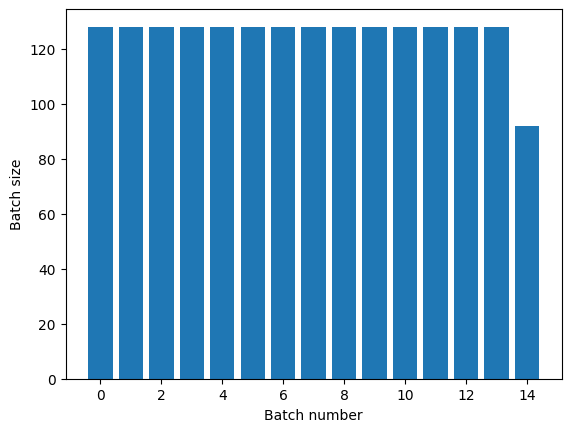If you need clear epoch separation, put Dataset.batch before the repeat:

titanic_batches = titanic_lines.batch(128).repeat(3)

plot_batch_sizes(titanic_batches)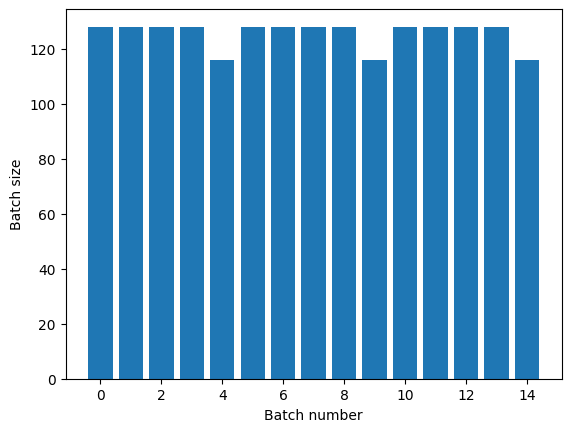If you would like to perform a custom computation (e.g. to collect statistics) at the end of each epoch then it's simplest to restart the dataset iteration on each epoch:

epochs = 3
dataset = titanic_lines.batch(128)

for epoch in range(epochs):
for batch in dataset:
print(batch.shape)
print("End of epoch: ", epoch)

(128,)
(128,)
(128,)
(128,)
(116,)
End of epoch:  0
(128,)
(128,)
(128,)
(128,)
(116,)
End of epoch:  1
(128,)
(128,)
(128,)
(128,)
(116,)
End of epoch:  2



### Randomly shuffling input data

The Dataset.shuffle() transformation maintains a fixed-size buffer and chooses the next element uniformly at random from that buffer.

Add an index to the dataset so you can see the effect:

lines = tf.data.TextLineDataset(titanic_file)
counter = tf.data.experimental.Counter()

dataset = tf.data.Dataset.zip((counter, lines))
dataset = dataset.shuffle(buffer_size=100)
dataset = dataset.batch(20)
dataset

<BatchDataset shapes: ((None,), (None,)), types: (tf.int64, tf.string)>


Since the buffer_size is 100, and the batch size is 20, the first batch contains no elements with an index over 120.

n,line_batch = next(iter(dataset))
print(n.numpy())

[ 29  39  94  10  81  85  74   3  91  53  87  17   1  64  54 107  20  63
116  62]



As with Dataset.batch the order relative to Dataset.repeat matters.

Dataset.shuffle doesn't signal the end of an epoch until the shuffle buffer is empty. So a shuffle placed before a repeat will show every element of one epoch before moving to the next:

dataset = tf.data.Dataset.zip((counter, lines))
shuffled = dataset.shuffle(buffer_size=100).batch(10).repeat(2)

print("Here are the item ID's near the epoch boundary:\n")
for n, line_batch in shuffled.skip(60).take(5):
print(n.numpy())

Here are the item ID's near the epoch boundary:

[469 560 614 431 557 558 618 616 571 532]
[464 403 509 589 365 610 596 428 600 605]
[539 467 163 599 624 545 552 548]
[92 30 73 51 72 71 56 83 32 46]
[ 74 106  75  78  26  21   3  54  80  50]


shuffle_repeat = [n.numpy().mean() for n, line_batch in shuffled]
plt.plot(shuffle_repeat, label="shuffle().repeat()")
plt.ylabel("Mean item ID")
plt.legend()

<matplotlib.legend.Legend at 0x7f79e43368d0>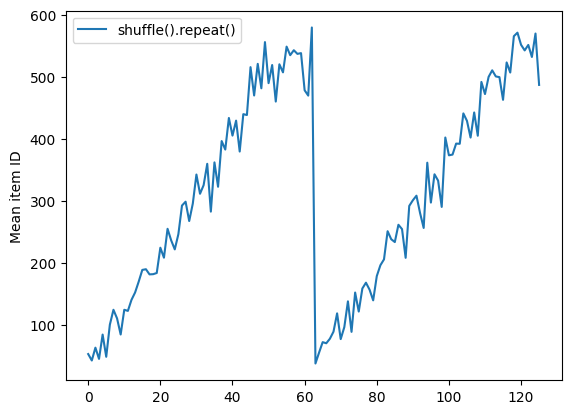But a repeat before a shuffle mixes the epoch boundaries together:

dataset = tf.data.Dataset.zip((counter, lines))
shuffled = dataset.repeat(2).shuffle(buffer_size=100).batch(10)

print("Here are the item ID's near the epoch boundary:\n")
for n, line_batch in shuffled.skip(55).take(15):
print(n.numpy())

Here are the item ID's near the epoch boundary:

[618 517  22  13 602 608  25 621  12 579]
[568 620 534 547 319   1  17 346  29 581]
[537 395 560  33 617 557 561 610 585 627]
[428 607  32   0 455 496 605 624   6 596]
[511 588  14 572   4  21  66 471  41  36]
[583 622  49  54 616  75  65  57  76   7]
[ 77  37 574 586  74  56  28 419  73   5]
[599  83  31  61  91 481 577  98  26  50]
[  2 625  85  23 606 102 550  20 612  15]
[ 24  63 592 615 388  39  45  51  68  97]
[ 46  34  67  55  79  94 548  47  72 436]
[127  89  30  90 126 601 360  87 104  52]
[ 59 130 106 584 118 265  48  70 597 121]
[626 105 135  10  38  53 132 136 101   9]
[512 542 109 154 108  42 114  11  58 166]


repeat_shuffle = [n.numpy().mean() for n, line_batch in shuffled]

plt.plot(shuffle_repeat, label="shuffle().repeat()")
plt.plot(repeat_shuffle, label="repeat().shuffle()")
plt.ylabel("Mean item ID")
plt.legend()

<matplotlib.legend.Legend at 0x7f79e42b60b8>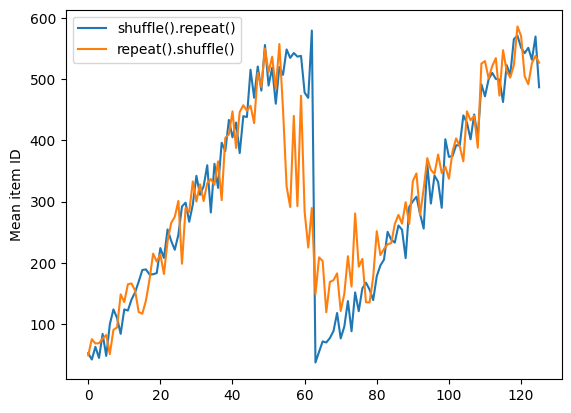## Preprocessing data

The Dataset.map(f) transformation produces a new dataset by applying a given function f to each element of the input dataset. It is based on the map() function that is commonly applied to lists (and other structures) in functional programming languages. The function f takes the tf.Tensor objects that represent a single element in the input, and returns the tf.Tensor objects that will represent a single element in the new dataset. Its implementation uses standard TensorFlow operations to transform one element into another.

This section covers common examples of how to use Dataset.map().

### Decoding image data and resizing it

When training a neural network on real-world image data, it is often necessary to convert images of different sizes to a common size, so that they may be batched into a fixed size.

Rebuild the flower filenames dataset:

list_ds = tf.data.Dataset.list_files(str(flowers_root/'*/*'))


Write a function that manipulates the dataset elements.

# Reads an image from a file, decodes it into a dense tensor, and resizes it
# to a fixed shape.
def parse_image(filename):
parts = tf.strings.split(filename, os.sep)
label = parts[-2]

image = tf.image.decode_jpeg(image)
image = tf.image.convert_image_dtype(image, tf.float32)
image = tf.image.resize(image, [128, 128])
return image, label


Test that it works.

file_path = next(iter(list_ds))
image, label = parse_image(file_path)

def show(image, label):
plt.figure()
plt.imshow(image)
plt.title(label.numpy().decode('utf-8'))
plt.axis('off')

show(image, label)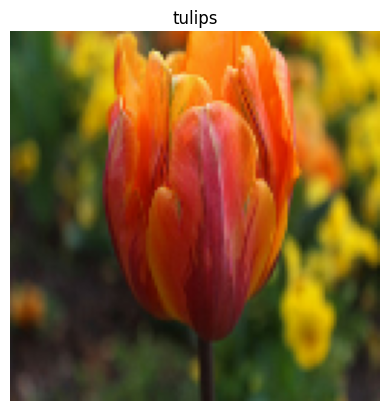Map it over the dataset.

images_ds = list_ds.map(parse_image)

for image, label in images_ds.take(2):
show(image, label)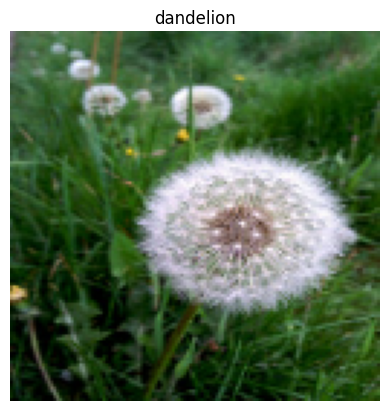### Applying arbitrary Python logic

For performance reasons, use TensorFlow operations for preprocessing your data whenever possible. However, it is sometimes useful to call external Python libraries when parsing your input data. You can use the tf.py_function() operation in a Dataset.map() transformation.

For example, if you want to apply a random rotation, the tf.image module only has tf.image.rot90, which is not very useful for image augmentation.

To demonstrate tf.py_function, try using the scipy.ndimage.rotate function instead:

import scipy.ndimage as ndimage

def random_rotate_image(image):
image = ndimage.rotate(image, np.random.uniform(-30, 30), reshape=False)
return image

image, label = next(iter(images_ds))
image = random_rotate_image(image)
show(image, label)

Clipping input data to the valid range for imshow with RGB data ([0..1] for floats or [0..255] for integers).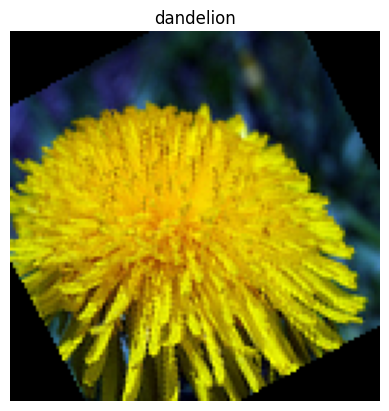To use this function with Dataset.map the same caveats apply as with Dataset.from_generator, you need to describe the return shapes and types when you apply the function:

def tf_random_rotate_image(image, label):
im_shape = image.shape
[image,] = tf.py_function(random_rotate_image, [image], [tf.float32])
image.set_shape(im_shape)
return image, label

rot_ds = images_ds.map(tf_random_rotate_image)

for image, label in rot_ds.take(2):
show(image, label)

Clipping input data to the valid range for imshow with RGB data ([0..1] for floats or [0..255] for integers).
Clipping input data to the valid range for imshow with RGB data ([0..1] for floats or [0..255] for integers).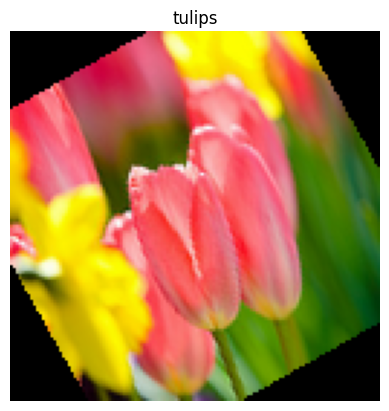### Parsing tf.Example protocol buffer messages

Many input pipelines extract tf.train.Example protocol buffer messages from a TFRecord format. Each tf.train.Example record contains one or more "features", and the input pipeline typically converts these features into tensors.

fsns_test_file = tf.keras.utils.get_file("fsns.tfrec", "https://storage.googleapis.com/download.tensorflow.org/data/fsns-20160927/testdata/fsns-00000-of-00001")
dataset = tf.data.TFRecordDataset(filenames = [fsns_test_file])
dataset

<TFRecordDatasetV2 shapes: (), types: tf.string>


You can work with tf.train.Example protos outside of a tf.data.Dataset to understand the data:

raw_example = next(iter(dataset))
parsed = tf.train.Example.FromString(raw_example.numpy())

feature = parsed.features.feature
raw_img = feature['image/encoded'].bytes_list.value
img = tf.image.decode_png(raw_img)
plt.imshow(img)
plt.axis('off')
_ = plt.title(feature["image/text"].bytes_list.value)raw_example = next(iter(dataset))

def tf_parse(eg):
example = tf.io.parse_example(
eg[tf.newaxis], {
'image/encoded': tf.io.FixedLenFeature(shape=(), dtype=tf.string),
'image/text': tf.io.FixedLenFeature(shape=(), dtype=tf.string)
})
return example['image/encoded'], example['image/text']

img, txt = tf_parse(raw_example)
print(txt.numpy())
print(repr(img.numpy()[:20]), "...")

b'Rue Perreyon'
b'\x89PNG\r\n\x1a\n\x00\x00\x00\rIHDR\x00\x00\x02X' ...


decoded = dataset.map(tf_parse)
decoded

<MapDataset shapes: ((), ()), types: (tf.string, tf.string)>

image_batch, text_batch = next(iter(decoded.batch(10)))
image_batch.shape

TensorShape()


### Time series windowing

For an end to end time series example see: Time series forecasting.

Time series data is often organized with the time axis intact.

Use a simple Dataset.range to demonstrate:

range_ds = tf.data.Dataset.range(100000)


Typically, models based on this sort of data will want a contiguous time slice.

The simplest approach would be to batch the data:

#### Using batch

batches = range_ds.batch(10, drop_remainder=True)

for batch in batches.take(5):
print(batch.numpy())

[0 1 2 3 4 5 6 7 8 9]
[10 11 12 13 14 15 16 17 18 19]
[20 21 22 23 24 25 26 27 28 29]
[30 31 32 33 34 35 36 37 38 39]
[40 41 42 43 44 45 46 47 48 49]



Or to make dense predictions one step into the future, you might shift the features and labels by one step relative to each other:

def dense_1_step(batch):
# Shift features and labels one step relative to each other.
return batch[:-1], batch[1:]

predict_dense_1_step = batches.map(dense_1_step)

for features, label in predict_dense_1_step.take(3):
print(features.numpy(), " => ", label.numpy())

[0 1 2 3 4 5 6 7 8]  =>  [1 2 3 4 5 6 7 8 9]
[10 11 12 13 14 15 16 17 18]  =>  [11 12 13 14 15 16 17 18 19]
[20 21 22 23 24 25 26 27 28]  =>  [21 22 23 24 25 26 27 28 29]



To predict a whole window instead of a fixed offset you can split the batches into two parts:

batches = range_ds.batch(15, drop_remainder=True)

def label_next_5_steps(batch):
return (batch[:-5],   # Take the first 5 steps
batch[-5:])   # take the remainder

predict_5_steps = batches.map(label_next_5_steps)

for features, label in predict_5_steps.take(3):
print(features.numpy(), " => ", label.numpy())

[0 1 2 3 4 5 6 7 8 9]  =>  [10 11 12 13 14]
[15 16 17 18 19 20 21 22 23 24]  =>  [25 26 27 28 29]
[30 31 32 33 34 35 36 37 38 39]  =>  [40 41 42 43 44]



To allow some overlap between the features of one batch and the labels of another, use Dataset.zip:

feature_length = 10
label_length = 5

features = range_ds.batch(feature_length, drop_remainder=True)
labels = range_ds.batch(feature_length).skip(1).map(lambda labels: labels[:-5])

predict_5_steps = tf.data.Dataset.zip((features, labels))

for features, label in predict_5_steps.take(3):
print(features.numpy(), " => ", label.numpy())

[0 1 2 3 4 5 6 7 8 9]  =>  [10 11 12 13 14]
[10 11 12 13 14 15 16 17 18 19]  =>  [20 21 22 23 24]
[20 21 22 23 24 25 26 27 28 29]  =>  [30 31 32 33 34]



#### Using window

While using Dataset.batch works, there are situations where you may need finer control. The Dataset.window method gives you complete control, but requires some care: it returns a Dataset of Datasets. See Dataset structure for details.

window_size = 5

windows = range_ds.window(window_size, shift=1)
for sub_ds in windows.take(5):
print(sub_ds)

<_VariantDataset shapes: (), types: tf.int64>
<_VariantDataset shapes: (), types: tf.int64>
<_VariantDataset shapes: (), types: tf.int64>
<_VariantDataset shapes: (), types: tf.int64>
<_VariantDataset shapes: (), types: tf.int64>



The Dataset.flat_map method can take a dataset of datasets and flatten it into a single dataset:

 for x in windows.flat_map(lambda x: x).take(30):
print(x.numpy(), end=' ')

WARNING:tensorflow:AutoGraph could not transform <function <lambda> at 0x7f79e44309d8> and will run it as-is.
Cause: could not parse the source code:

for x in windows.flat_map(lambda x: x).take(30):

This error may be avoided by creating the lambda in a standalone statement.

To silence this warning, decorate the function with @tf.autograph.experimental.do_not_convert
WARNING: AutoGraph could not transform <function <lambda> at 0x7f79e44309d8> and will run it as-is.
Cause: could not parse the source code:

for x in windows.flat_map(lambda x: x).take(30):

This error may be avoided by creating the lambda in a standalone statement.

To silence this warning, decorate the function with @tf.autograph.experimental.do_not_convert
0 1 2 3 4 1 2 3 4 5 2 3 4 5 6 3 4 5 6 7 4 5 6 7 8 5 6 7 8 9


In nearly all cases, you will want to .batch the dataset first:

def sub_to_batch(sub):
return sub.batch(window_size, drop_remainder=True)

for example in windows.flat_map(sub_to_batch).take(5):
print(example.numpy())

[0 1 2 3 4]
[1 2 3 4 5]
[2 3 4 5 6]
[3 4 5 6 7]
[4 5 6 7 8]



Now, you can see that the shift argument controls how much each window moves over.

Putting this together you might write this function:

def make_window_dataset(ds, window_size=5, shift=1, stride=1):
windows = ds.window(window_size, shift=shift, stride=stride)

def sub_to_batch(sub):
return sub.batch(window_size, drop_remainder=True)

windows = windows.flat_map(sub_to_batch)
return windows


ds = make_window_dataset(range_ds, window_size=10, shift = 5, stride=3)

for example in ds.take(10):
print(example.numpy())

[ 0  3  6  9 12 15 18 21 24 27]
[ 5  8 11 14 17 20 23 26 29 32]
[10 13 16 19 22 25 28 31 34 37]
[15 18 21 24 27 30 33 36 39 42]
[20 23 26 29 32 35 38 41 44 47]
[25 28 31 34 37 40 43 46 49 52]
[30 33 36 39 42 45 48 51 54 57]
[35 38 41 44 47 50 53 56 59 62]
[40 43 46 49 52 55 58 61 64 67]
[45 48 51 54 57 60 63 66 69 72]



Then it's easy to extract labels, as before:

dense_labels_ds = ds.map(dense_1_step)

for inputs,labels in dense_labels_ds.take(3):
print(inputs.numpy(), "=>", labels.numpy())

[ 0  3  6  9 12 15 18 21 24] => [ 3  6  9 12 15 18 21 24 27]
[ 5  8 11 14 17 20 23 26 29] => [ 8 11 14 17 20 23 26 29 32]
[10 13 16 19 22 25 28 31 34] => [13 16 19 22 25 28 31 34 37]



### Resampling

When working with a dataset that is very class-imbalanced, you may want to resample the dataset. tf.data provides two methods to do this. The credit card fraud dataset is a good example of this sort of problem.

zip_path = tf.keras.utils.get_file(
fname='creditcard.zip',
extract=True)

csv_path = zip_path.replace('.zip', '.csv')

Downloading data from https://storage.googleapis.com/download.tensorflow.org/data/creditcard.zip
69156864/69155632 [==============================] - 3s 0us/step


creditcard_ds = tf.data.experimental.make_csv_dataset(
csv_path, batch_size=1024, label_name="Class",
# Set the column types: 30 floats and an int.
column_defaults=[float()]*30+[int()])


Now, check the distribution of classes, it is highly skewed:

def count(counts, batch):
features, labels = batch
class_1 = labels == 1
class_1 = tf.cast(class_1, tf.int32)

class_0 = labels == 0
class_0 = tf.cast(class_0, tf.int32)

counts['class_0'] += tf.reduce_sum(class_0)
counts['class_1'] += tf.reduce_sum(class_1)

return counts

counts = creditcard_ds.take(10).reduce(
initial_state={'class_0': 0, 'class_1': 0},
reduce_func = count)

counts = np.array([counts['class_0'].numpy(),
counts['class_1'].numpy()]).astype(np.float32)

fractions = counts/counts.sum()
print(fractions)

[0.9957 0.0043]



A common approach to training with an imbalanced dataset is to balance it. tf.data includes a few methods which enable this workflow:

#### Datasets sampling

One approach to resampling a dataset is to use sample_from_datasets. This is more applicable when you have a separate data.Dataset for each class.

Here, just use filter to generate them from the credit card fraud data:

negative_ds = (
creditcard_ds
.unbatch()
.filter(lambda features, label: label==0)
.repeat())
positive_ds = (
creditcard_ds
.unbatch()
.filter(lambda features, label: label==1)
.repeat())

WARNING:tensorflow:AutoGraph could not transform <function <lambda> at 0x7f79e43ce510> and will run it as-is.
Cause: could not parse the source code:

.filter(lambda features, label: label==0)

This error may be avoided by creating the lambda in a standalone statement.

To silence this warning, decorate the function with @tf.autograph.experimental.do_not_convert
WARNING: AutoGraph could not transform <function <lambda> at 0x7f79e43ce510> and will run it as-is.
Cause: could not parse the source code:

.filter(lambda features, label: label==0)

This error may be avoided by creating the lambda in a standalone statement.

To silence this warning, decorate the function with @tf.autograph.experimental.do_not_convert
WARNING:tensorflow:AutoGraph could not transform <function <lambda> at 0x7f79e43ceae8> and will run it as-is.
Cause: could not parse the source code:

.filter(lambda features, label: label==1)

This error may be avoided by creating the lambda in a standalone statement.

To silence this warning, decorate the function with @tf.autograph.experimental.do_not_convert
WARNING: AutoGraph could not transform <function <lambda> at 0x7f79e43ceae8> and will run it as-is.
Cause: could not parse the source code:

.filter(lambda features, label: label==1)

This error may be avoided by creating the lambda in a standalone statement.

To silence this warning, decorate the function with @tf.autograph.experimental.do_not_convert


for features, label in positive_ds.batch(10).take(1):
print(label.numpy())

[1 1 1 1 1 1 1 1 1 1]



To use tf.data.experimental.sample_from_datasets pass the datasets, and the weight for each:

balanced_ds = tf.data.experimental.sample_from_datasets(
[negative_ds, positive_ds], [0.5, 0.5]).batch(10)


Now the dataset produces examples of each class with 50/50 probability:

for features, labels in balanced_ds.take(10):
print(labels.numpy())

[0 1 1 1 1 0 0 1 0 1]
[0 0 0 0 1 1 1 0 0 1]
[1 0 1 0 1 1 0 0 1 0]
[0 0 0 0 1 0 1 1 0 0]
[0 1 1 1 0 1 1 1 1 1]
[0 1 0 1 1 0 1 1 0 1]
[0 0 1 1 1 0 1 1 1 1]
[1 1 0 0 1 1 0 1 1 0]
[0 1 0 0 1 0 0 0 0 0]
[0 1 1 1 0 1 0 1 1 0]



#### Rejection resampling

One problem with the above experimental.sample_from_datasets approach is that it needs a separate tf.data.Dataset per class. Using Dataset.filter works, but results in all the data being loaded twice.

The data.experimental.rejection_resample function can be applied to a dataset to rebalance it, while only loading it once. Elements will be dropped from the dataset to achieve balance.

data.experimental.rejection_resample takes a class_func argument. This class_func is applied to each dataset element, and is used to determine which class an example belongs to for the purposes of balancing.

The elements of creditcard_ds are already (features, label) pairs. So the class_func just needs to return those labels:

def class_func(features, label):
return label


The resampler also needs a target distribution, and optionally an initial distribution estimate:

resampler = tf.data.experimental.rejection_resample(
class_func, target_dist=[0.5, 0.5], initial_dist=fractions)


The resampler deals with individual examples, so you must unbatch the dataset before applying the resampler:

resample_ds = creditcard_ds.unbatch().apply(resampler).batch(10)

WARNING:tensorflow:From /tmpfs/src/tf_docs_env/lib/python3.6/site-packages/tensorflow/python/data/experimental/ops/resampling.py:156: Print (from tensorflow.python.ops.logging_ops) is deprecated and will be removed after 2018-08-20.
Instructions for updating:
Use tf.print instead of tf.Print. Note that tf.print returns a no-output operator that directly prints the output. Outside of defuns or eager mode, this operator will not be executed unless it is directly specified in session.run or used as a control dependency for other operators. This is only a concern in graph mode. Below is an example of how to ensure tf.print executes in graph mode:



The resampler returns creates (class, example) pairs from the output of the class_func. In this case, the example was already a (feature, label) pair, so use map to drop the extra copy of the labels:

balanced_ds = resample_ds.map(lambda extra_label, features_and_label: features_and_label)


Now the dataset produces examples of each class with 50/50 probability:

for features, labels in balanced_ds.take(10):
print(labels.numpy())

[1 0 1 1 0 0 0 0 0 0]
[1 0 1 0 1 1 0 1 0 1]
[0 1 1 0 0 1 0 0 0 0]
[1 0 1 0 0 1 0 1 0 0]
[1 1 1 1 0 0 0 0 0 1]
[1 0 1 1 1 0 1 0 1 1]
[0 0 1 1 1 1 0 0 1 1]
[0 0 1 0 0 1 1 1 0 1]
[1 0 0 0 1 0 0 0 0 0]
[0 0 0 0 1 0 0 0 1 1]



## Iterator Checkpointing

Tensorflow supports taking checkpoints so that when your training process restarts it can restore the latest checkpoint to recover most of its progress. In addition to checkpointing the model variables, you can also checkpoint the progress of the dataset iterator. This could be useful if you have a large dataset and don't want to start the dataset from the beginning on each restart. Note however that iterator checkpoints may be large, since transformations such as shuffle and prefetch require buffering elements within the iterator.

To include your iterator in a checkpoint, pass the iterator to the tf.train.Checkpoint constructor.

range_ds = tf.data.Dataset.range(20)

iterator = iter(range_ds)
ckpt = tf.train.Checkpoint(step=tf.Variable(0), iterator=iterator)
manager = tf.train.CheckpointManager(ckpt, '/tmp/my_ckpt', max_to_keep=3)

print([next(iterator).numpy() for _ in range(5)])

save_path = manager.save()

print([next(iterator).numpy() for _ in range(5)])

ckpt.restore(manager.latest_checkpoint)

print([next(iterator).numpy() for _ in range(5)])

[0, 1, 2, 3, 4]
[5, 6, 7, 8, 9]
[5, 6, 7, 8, 9]



## Using tf.data with tf.keras

The tf.keras API simplifies many aspects of creating and executing machine learning models. Its .fit() and .evaluate() and .predict() APIs support datasets as inputs. Here is a quick dataset and model setup:

train, test = tf.keras.datasets.fashion_mnist.load_data()

images, labels = train
images = images/255.0
labels = labels.astype(np.int32)

fmnist_train_ds = tf.data.Dataset.from_tensor_slices((images, labels))
fmnist_train_ds = fmnist_train_ds.shuffle(5000).batch(32)

model = tf.keras.Sequential([
tf.keras.layers.Flatten(),
tf.keras.layers.Dense(10)
])

loss=tf.keras.losses.SparseCategoricalCrossentropy(from_logits=True),
metrics=['accuracy'])


Passing a dataset of (feature, label) pairs is all that's needed for Model.fit and Model.evaluate:

model.fit(fmnist_train_ds, epochs=2)

Epoch 1/2
WARNING:tensorflow:Layer flatten is casting an input tensor from dtype float64 to the layer's dtype of float32, which is new behavior in TensorFlow 2.  The layer has dtype float32 because its dtype defaults to floatx.

If you intended to run this layer in float32, you can safely ignore this warning. If in doubt, this warning is likely only an issue if you are porting a TensorFlow 1.X model to TensorFlow 2.

To change all layers to have dtype float64 by default, call tf.keras.backend.set_floatx('float64'). To change just this layer, pass dtype='float64' to the layer constructor. If you are the author of this layer, you can disable autocasting by passing autocast=False to the base Layer constructor.

1875/1875 [==============================] - 3s 2ms/step - loss: 0.5973 - accuracy: 0.7969
Epoch 2/2
1875/1875 [==============================] - 3s 2ms/step - loss: 0.4629 - accuracy: 0.8407

<tensorflow.python.keras.callbacks.History at 0x7f79e43d8710>


If you pass an infinite dataset, for example by calling Dataset.repeat(), you just need to also pass the steps_per_epoch argument:

model.fit(fmnist_train_ds.repeat(), epochs=2, steps_per_epoch=20)

Epoch 1/2
20/20 [==============================] - 0s 2ms/step - loss: 0.4800 - accuracy: 0.8594
Epoch 2/2
20/20 [==============================] - 0s 2ms/step - loss: 0.4529 - accuracy: 0.8391

<tensorflow.python.keras.callbacks.History at 0x7f79e43d84a8>


For evaluation you can pass the number of evaluation steps:

loss, accuracy = model.evaluate(fmnist_train_ds)
print("Loss :", loss)
print("Accuracy :", accuracy)

1875/1875 [==============================] - 3s 2ms/step - loss: 0.4386 - accuracy: 0.8508
Loss : 0.4385797381401062
Accuracy : 0.8507500290870667



For long datasets, set the number of steps to evaluate:

loss, accuracy = model.evaluate(fmnist_train_ds.repeat(), steps=10)
print("Loss :", loss)
print("Accuracy :", accuracy)

10/10 [==============================] - 0s 2ms/step - loss: 0.3737 - accuracy: 0.8656
Loss : 0.37370163202285767
Accuracy : 0.8656250238418579



The labels are not required in when calling Model.predict.

predict_ds = tf.data.Dataset.from_tensor_slices(images).batch(32)
result = model.predict(predict_ds, steps = 10)
print(result.shape)

(320, 10)



But the labels are ignored if you do pass a dataset containing them:

result = model.predict(fmnist_train_ds, steps = 10)
print(result.shape)

(320, 10)